United States Department of LaborThe .gov means it's official.
Federal government websites often end in .gov or .mil. Before sharing sensitive information, make sure you're on a federal government site.The site is secure.
The https:// ensures that you are connecting to the official website and that any information you provide is encrypted and transmitted securely.

Consumer Expenditure Surveys

### Consumer Expenditure Survey Program How does the variability of Consumer Expenditure data impact your analysis?

Aaron Cobet
Bureau of Labor Statistics
February 3, 2017

The Consumer Expenditure Surveys Program (CE) collects data on all reported expenditures and most types of income in the United States. CE provides these data by geographic region and many demographic characteristics.

However, some CE estimates may not be precise enough for your needs because CE may only provide a wide range of possible values rather than an exact data point.1 This report addresses these related questions:

### What creates variability in sample data?

Data that are based on surveys have some degree of variability, which means that the reported value is an approximation of the actual value. Generally, the reported value is an average of the sampled observations. The size of the variability dependds on two factors.

• #### Sample size

• The sample size refers to the number of observations from the whole. Generally, the large the sample is the smaller the variability (See chart 1).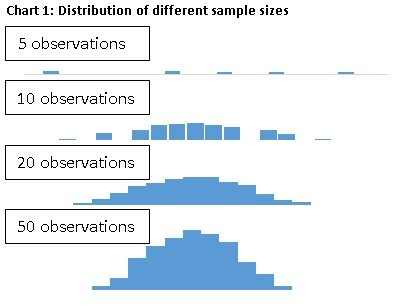• #### Dispersion of the sample

• Dispersion refers to how closely or widely the dollar amounts for an expenditure are dispersed. Generally, the larger the dispersion the larger the variability. In chart 2, the blue sample has a smaller dispersion than the red sample. Thus, the variability of the blue sample is smaller than the variability of the red sample.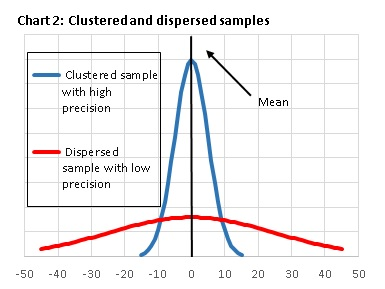### How does CE measure variability?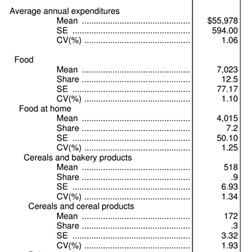The CE tables provide two measures of variability:

• #### Standard error (SE)

• A SE measures the absolute dispersion of an estimate. For CE, SE shows how close the dollar average of the sample is to the average of the populations. CE calculates a modified version of the standard SE formula due to its sample design.2

• #### Coefficient of variation (CV)

• A CV measures the relative dispersion of an estimate. CVs are expressed as percentages, dividing an estimate's standard deviation (SD) by the estimate. The CV is also known as relative standard deviation (RSD).

### How does variability affect you?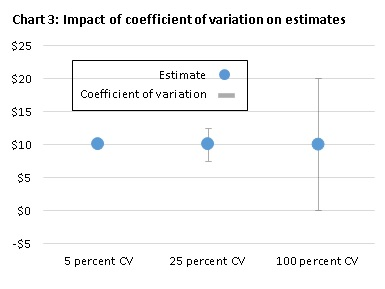Variability limits your ability to use the data with certainty. Measures of variability inform you about the range of possible values for a particular data point. Generally, the larger that range is the lower your confidence in the data point.3

Chart 3 shows the effect of increasing ranges on the range of possible values with three different CVs:

• 5 percent
• 25 percent
• 100 percent

The range of the possible estimates expands with a 5 percent CV to values between \$9.50 and \$10.50, with a 25 percent CV to \$12.50 to \$7.50, and with a 100 percent CV to \$0.00 and \$20.00. The third example shows how a large CV may affect your analysis.

The CE tables show the degree of precision with CVs. CE staff strongly recommend using estimates with CVs that are below 25 percent. CV's of 25 percent allow you to state with certainty that the actual value is no larger or smaller than 25 percent of the estimate.4 However, there is no rule about what precision is sufficient for your analysis. For more information on this topic, see when can you compare two data points with confidence?

### When can you compare two data points with confidence?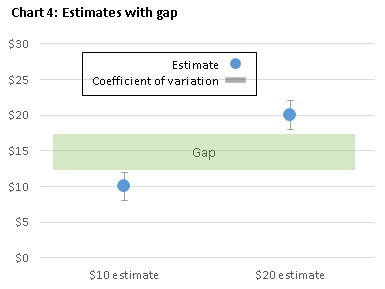You can determine which data point is larger if there is a gap between two points after you consider their variation or CV. By contrast you cannot determine which estimate is larger if the two data points with their CVs overlap.

Charts 4 and 5 provide examples for both scenarios. Both charts show on the left a \$10 estimate and on the right a \$20 estimate.

In chart 4, the two estimates have a gap after taking their CV into account. In this case, you can determine that the \$10 estimate is definitively smaller than the \$20 estimate because the biggest possible value of the \$10 estimate is \$12.50 and the smallest possible value of the \$20 estimate is to \$17.50. Since \$12.50 is smaller than \$17.50, the \$10 estimate is smaller than the \$20 estimate.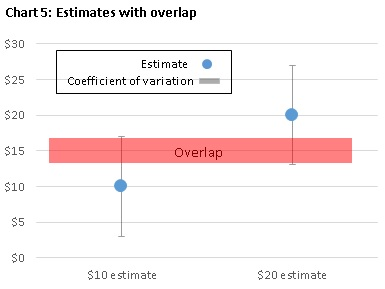In contrast, chart 5 shows two data points that have an overlap after taking their CV into account. In this case, you cannot determine with certainty that the \$10 estimate is smaller than the \$20 estimate because the biggest possible value of the \$10 estimate is \$17, and the smallest possible value of the \$20 estimate is \$13. Since \$17 is larger than \$13, the \$10 estimate might be larger than the \$20 estimate.

### How can you decrease the variability of CE estimates?

You can increase the precision of estimates or decrease its range of possible values by increasing the number of observations in your sample. You can increase the sample size by expanding your research question and dropping aspects from consideration that are less important to your analysis.

Let's say you want to look at expenses for men's shirts in the Northeast by households earning \$50,000 to \$69,999 in 2015. However, you determine that the data are not precise enough for your analysis. You could drop the least important aspect until the precision meets your needs.

For CE data, you may be able to increase your sample with these changes:

• Enlarge the region, if you assume that buying habits are sufficiently alike in different regions. For example, if the Northeast has too few observations, maybe include the Midwest. This method is possible with CE tables and Public-use Microdata (PUMD) .
• Increase the income range, if you assume that households with a broader income range follow an adequately similar spending pattern. For example, you could consider households earning between \$30,000 and \$69,999. This method is possible with tables and PUMD.
• Broaden the item category, if you assume that the broader category behaves sufficiently similar. For example, you may look at clothes instead of just men's shirts. This method is possible with tables and PUMD.
• Expand the time period, if you assume that expenses do not change significantly over time. For example if the data for one year are not precise enough, consider aggregating two years into one data point. This method is possible with PUMD.

You can use these methods to a varying degree with two CE products:

• CE tables provide some flexibility with respect to the item's detail, income groups, age groups, and a few other characteristics.
• CE Public-use Microdata (PUMD) provides you the maximum flexibility to change aspects and add years. However to use PUMD, you need to calculate estimates with a statistical software, such as SAS or R. For more information on PUMD, see the Getting Started Guide.

### What additional resources does CE provide?

For additional information on this issue, CE provides these resources:

• The methodology that CE uses to calculate the standard error is described in the "Standard errors in the 2015 Consumer Expenditure Survey" by David Swanson at https://www.bls.gov/cex/ce_se_2015.pdf.
• A summary of the precision of CE data is presented in the "Calculation Precision" section of the Handbook of Methods on page 18 at https://www.bls.gov/opub/hom/cex/pdf/cex.pdf.
• How can you increase the precision of estimates by pooling data from several years is described in the"estimation procedure" section of the Interview Survey Documentation at https://www.bls.gov/cex/2015/csxintvw.pdf.

#### Notes

1 CE data can be used to derive two types of estimates. CE tables and the database provide weighted population estimates, which refer to estimates that have been weighted to be representative of the entire population. In addition, users can use Public-use Microdata to calculate not only other weighted population estimates, but also unweighted sample estimates, which provide an estimate for the respondents who made this expenditure or had this income type.

2 CE does not calculate the standard error with the standard formula because this formula requires that the sample is collected as a simple random sample. However, the CE surveys draw stratified random samples of geographic areas and a systematic sample of households within the selected areas. To estimate an unbiased variance with this sampling method, CE uses the balanced repeated replication (BRR) method. For more information on this issue, see "Standard Errors in the 2015 Consumer Expenditure Survey".

3 This range is called confidence interval, which measures the probability that a population parameter falls between two values.

4 CE estimates do not provide 100 percent certainty. For more information on the degree of certainty of CE estimates, see Standard Errors in the 2015 Consumer Expenditure Survey by David Swanson.